# Python – Tkinter askquestion Dialog

In Python, There Are Serval Libraries for Graphical User Interface. Tkinter is one of them that is most useful. It is a standard interface. Tkinter is easy to use and provides serval functions for building efficient applications. In Every Application, we need some Message to Display like “Do You Want To Close ” or showing any warning or Something information. For this Tkinter provide library like messagebox. By using the message box library we can show serval Information, Error, Warning, Cancelation ETC in the from of Message-Box. It has Different the message box for a different purpose.

1. showinfo() – To display some important information .
2. showwarning() – To display some type of Warning.
3. showerror() –To display some Error Message.
4. askquestion() – To display dilog box that ask with two options YES or NO.
5. askokcancel() – To display dilog box that ask with two options OK or CANCEL.
6. askretrycancel() – To display dilog box that ask with two options RETRY or CANCEL.
7. askyesnocancel() – To display dilog box that ask with three options YES or NO or CANCEL.

Syntax of the Message-Box Functions:

`  messagebox.name_of_function(Title, Message, [, options]); `
1. name_of_function – Function name that which we want to use .
2. Title – Message Box’s Title.
3. Message – Message that you want to show in dailog.
4. Options –To configure the options .

This function is used to ask the questions to user. that have only two options YES or NO.
Application of this function –

1. We can use this to ask the user that User want’s to continue.
2. We can use this to ask the user that User wanted to Submit or not.

Syntax:

`messagebox.askfunction((Title, Message, [, options]); `

Example:

 `from` `tkinter ``import` `*` `from` `tkinter ``import` `messagebox ` `  `  `     `  `# object of TK() ` `main ``=` `Tk() ` ` `  `# function to use the  ` `# askquestion() function ` `def` `Submit():  ` `    ``messagebox.askquestion(``"Form"``, ` `                           ``"Do you want to Submit"``) ` `     `  `# setting geometry of window  ` `# instance ` `main.geometry(``"100x100"``) ` ` `  `# creating Window ` `B1 ``=` `Button(main, text ``=` `"Submit"``, command ``=` `Submit)  ` ` `  `# Button positioning  ` `B1.pack()    ` ` `  `# infinte loop till close ` `main.mainloop()   `

Output: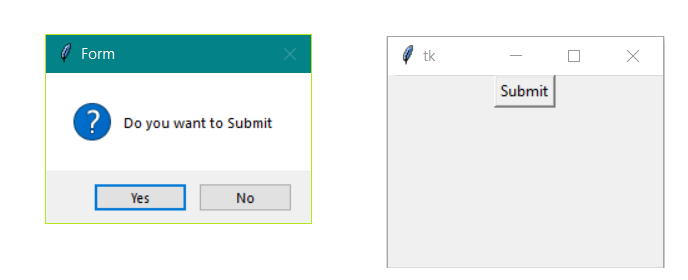1. Importing the Libraries
To use the GUI functionality in python we have to import the libraries . In the first line we are importing tkinter and second line we importing messagebox library

```from tkinter import *
from tkinter import messagebox
```

2. Main window instance
We have to create an instance or object for thw window ot TK(); Tk() is function of tkinter that create a window that can be refered from main variable

`main = Tk()  `

3. Set dimenstion
we set the dimention of the window we can set it by various way.in this we are setting is by geometry() function of size “100X100”.

`top.geometry("100x100")  `

4. Applying other widget and function
In our example we create a method named Submit and call the askquestion() and Creating Button and setting it by Pack() function

```def Submit():
messagebox.askquestion("Form", "Do you want to Submit")

main.geometry("100x100")
B1 = Button(main, text = "Submit", command = Submit)
B1.pack()
```

5. mainloop()
This method can be used when all the code is ready to execute.It runs the INFINITE Loop used to run the application.A window will open until the close button is pressed.

ICONS that We can use in Options

1. Error
2. Info
3. Warning
4. Question

We can change the icon of the dialog box. The type of icon that we want to use just depends on the application’s need. we have four icons.
Error

` messagebox.function_name(Title, Message, icon='error')`

Example-

 `# illustration of icon - Error ` `from` `tkinter ``import` `*` `from` `tkinter ``import` `messagebox ` ` `  `main ``=` `Tk() ` ` `  `def` `check(): ` `   ``messagebox.askquestion(``"Form"``,  ` `                          ``"Is your name correct?"``,  ` `                          ``icon ``=``'error'``) ` ` `  `main.geometry(``"100x100"``) ` `B1 ``=` `Button(main, text ``=` `"check"``, command ``=` `check) ` `B1.pack() ` ` `  `main.mainloop() `

Output: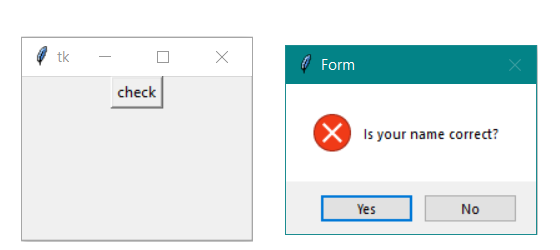info

` messagebox.function_name(Title, Message, icon='info')`

Example-

 `# illustration of icon - Info ` `from` `tkinter ``import` `*` `from` `tkinter ``import` `messagebox ` ` `  `main ``=` `Tk() ` ` `  `def` `check(): ` `   ``messagebox.askquestion(``"Form"``, ` `                          ``"do you want to continue"``,  ` `                          ``icon ``=``'info'``) ` ` `  `main.geometry(``"100x100"``) ` `B1 ``=` `Button(main, text ``=` `"check"``, command ``=` `check) ` `B1.pack() ` ` `  `main.mainloop() `

Output: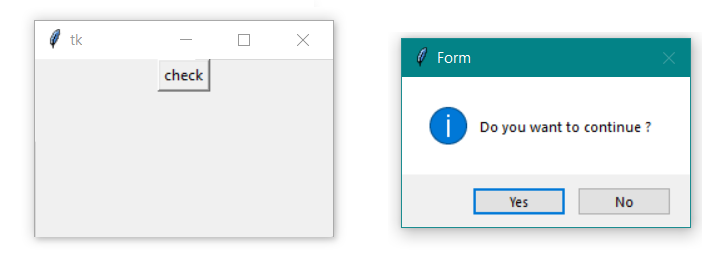Question

` messagebox.function_name(Title, Message, icon='question')`

Example-

 `# illustration of icon - question ` `from` `tkinter ``import` `*` `from` `tkinter ``import` `messagebox ` ` `  `main ``=` `Tk() ` ` `  `def` `check(): ` `   ``messagebox.askquestion(``"Form"``, ` `                          ``"are you 18+"``,  ` `                          ``icon ``=``'question'``) ` ` `  `main.geometry(``"100x100"``) ` `B1 ``=` `Button(main, text ``=` `"check"``, command ``=` `check) ` `B1.pack() ` ` `  `main.mainloop() `

Output: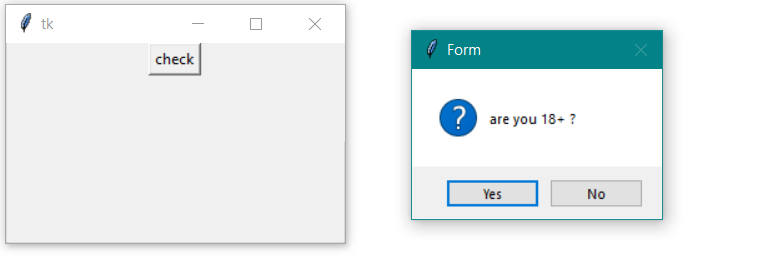Warning

`messagebox.function_name(Title, Message, icon='warning')`

Example-

 `# illustration of icon - Warning ` `from` `tkinter ``import` `*` `from` `tkinter ``import` `messagebox ` ` `  `main ``=` `Tk() ` ` `  `def` `check(): ` `   ``messagebox.askquestion(``"Form"``, ` `                          ``"Gender is empty?"``, ` `                          ``icon ``=``'warning'``) ` ` `  `main.geometry(``"100x100"``) ` `B1 ``=` `Button(main, text ``=` `"check"``, command ``=` `check) ` `B1.pack() ` ` `  `main.mainloop() `

Output: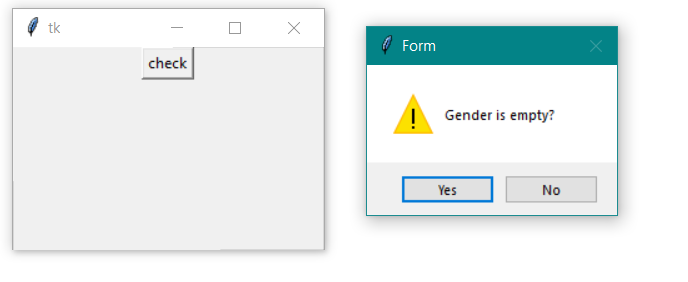My Personal Notes arrow_drop_upCheck out this Author's contributed articles.

If you like GeeksforGeeks and would like to contribute, you can also write an article using contribute.geeksforgeeks.org or mail your article to contribute@geeksforgeeks.org. See your article appearing on the GeeksforGeeks main page and help other Geeks.

Please Improve this article if you find anything incorrect by clicking on the "Improve Article" button below.

Improved By : Akanksha_Rai

Article Tags :

Be the First to upvote.

Please write to us at contribute@geeksforgeeks.org to report any issue with the above content.